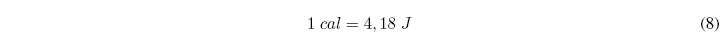What is internal energy? To understand the concept of internal energy, let's consider a gas. Each gas molecule has four types of energy:
Translational energy is kinetic energy due to the movement of the molecule.
Vibrational energy is the vibration energy of the atoms around the bond that unites them.
Rotational energy, due to the rotation of the molecule.
Electronic energy, produced by electronic transitions. It only matters at elevated temperatures.
The sum of these four types of energy gives us the total energy of a molecule. Adding the energy of all the molecules we will obtain the internal energy of the gas. Note that a monatomic gas has only translational and electronic energy since it cannot rotate or vibrate.

How can we change the internal energy of a system? The change of internal energy of a system is produced by exchanges of heat and/or work. When we do work or add heat to a system, we increase its internal energy. If the system loses heat or does work on the surroundings, its internal energy decreases.
The first law of thermodynamics can be written as follows:Where $\Delta U$ is the change in internal energy, q is the work absorbed $(q>0)$ or released by the system (q<0) and w represents the work done on the system w>0 or by the system over the environment $(w<0)$.
The first principle tells us that energy is conserved, it is the principle of conservation of energy. The internal energy of a system can be transformed into heat or work. Heat and work can be converted into internal energy, but it will always be true that the sum of heat and work is equal to the change in internal energy.The internal energy is a function of state. For any process, the change in internal energy only depends on the initial and final states of the system and is independent of the path followed. For a process in which the system goes from state 1 to state 2, the change in internal energy is given by the expression:In addition, in any cyclic process, the internal energy variation is null.

$\Delta U=0$ , cyclic process.

Unlike internal energy, heat and work are not state functions and in order to calculate them it is necessary to know the trajectory followed by the system throughout the entire process that takes us from point 1 to point 2.

Both heat, work, and internal energy have units of Joules. Another widely used unit of energy is the calorie.For pure substances, the molar internal energy $\bar{U}=U/n$ is defined, where n is the number of moles of the pure substance.